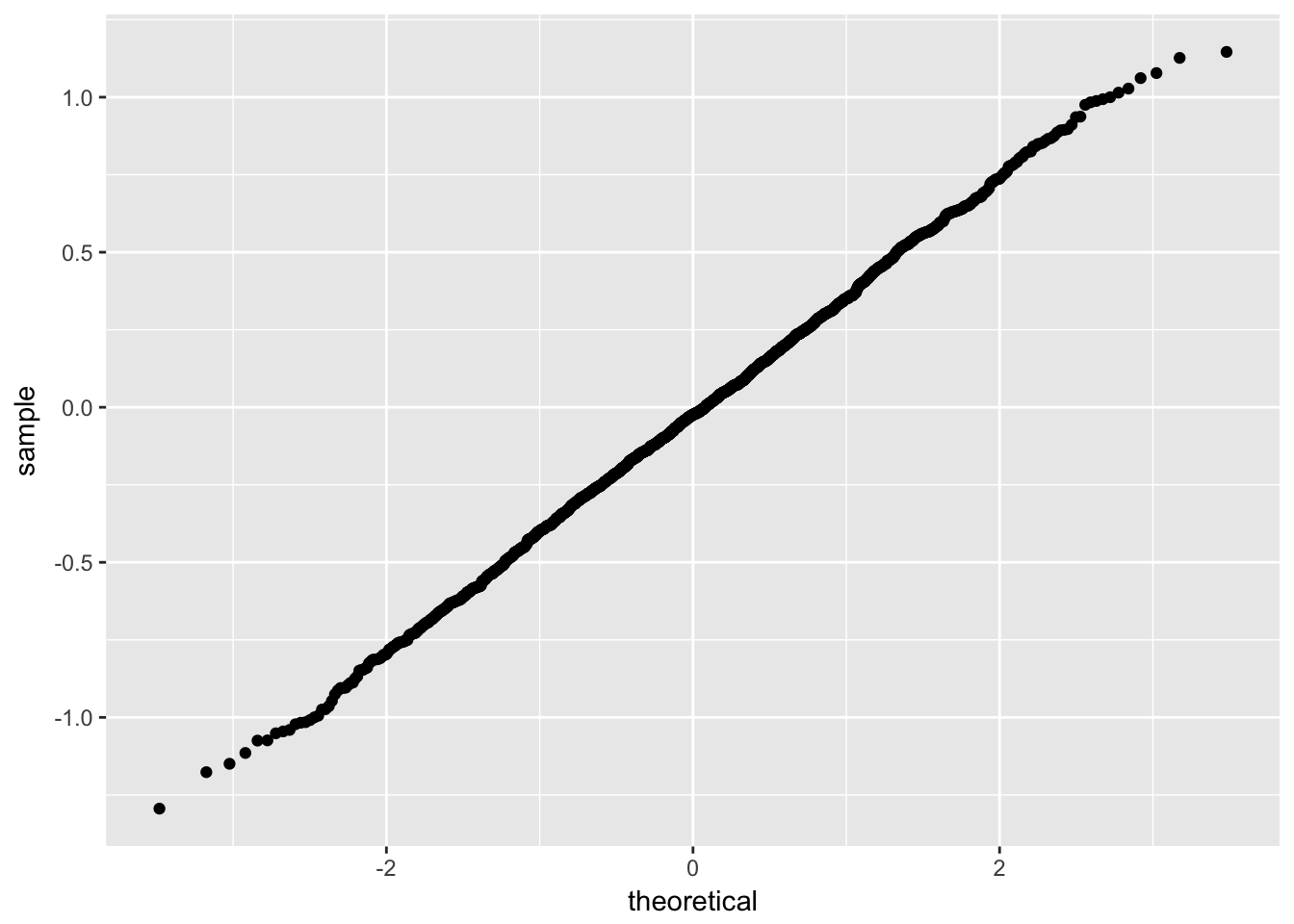## Definition

It is used to estimate the population mean $$\mu$$

$\overline{X}_n=\frac{\sum_{i=1}^{n} X_i}{n}$

## Bias

$bias(\overline{X}_n) = E[\overline{X}_n] - \mu = 0$

• Demonstration

$$E[\overline{X}_n] = \frac{1}{n} \sum_{i} E[X_i] = \frac{1}{n} n E[X_i]= E[X_i]= \mu$$

## Standard error

$se = \frac{\sigma}{\sqrt{n}}$ where $$\sigma^2$$ is the population variance.

### Demonstration

$V[\overline{X}_n]=V(\frac{1}{n}\sum_i X_i) = \frac{1}{n^2} \sum_i V(X_i) = \frac{1}{n^2} n \sigma^2 = \frac{\sigma^2}{n}$

## Distribution

### For a normal distributed random variable (normal parent distribution)

If $$X_1,\dotsc,X_n$$ are normally distributed, then $$\overline{X}_n$$ is distributed $$N(\mu,\frac{\sigma^2}{n})$$

### Simulation example

library(tidyverse)

M <- 1:2000
n <- 1:7
mu <- 100
sigma <- 16

sim_sample_mean <- crossing(M, n) %>%
group_by(M, n) %>%
mutate(x = rnorm(1, mu, sigma),
z = (x - mu)/sigma) %>%
group_by(M) %>%
summarise(sample_mean_x = mean(x),
sample_mean_z = mean(z))

ggplot(sim_sample_mean, aes(sample = sample_mean_z)) +
stat_qq()### For a large n (asymptotic normality, central limit theorem)

$$X_1,\dotsc,X_n$$ for large $$n$$, $$\overline{X}_n$$ is distributed $$N(\mu,\frac{\sigma^2}{n})$$

We say that $$\overline{X}_n$$ is asymptotically normal. That means that probability statements about the sample mean can be approximated using a normal distribution.

## Consistency (law of large numbers)

$$\overline{X}_n$$ is consistent because it converges in probability to $$\mu$$.